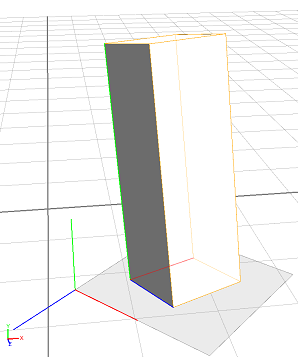# convert 函数

### 语法

• float convert(coordinate, fromSystem, toSystem, type, x, y, z)
• float[] convert(fromSystem, toSystem, type, coordinates)

### 参数

1. coordinate - 选择器
{x | y | z} - 要转换的坐标组件。
2. fromSystem - 选择器
{scope | pivot | object | world} - 要转换的坐标系。
3. toSystem - 选择器
{scope | pivot | object | world} - 要转换到的坐标系。
4. type - 选择器
{pos | orient} - 将 coordinates 数组或 (x, y, z) 元组的解释选择为坐标或角度。
5. x, y, z - 浮点型

coordinates - float[]

fromSystem 中要转换为 toSystem 的位置（坐标）/方向（角度，以度为单位）。

### 描述

convert 函数用于在不同坐标系之间转换位置和方向。

### 示例

 ``````Init --> extrude(3) t('0.2, 0, '0.7) s('0.5, '1, '0.5) r(-10, 70, 0) // position of scope origin in world coordinates print(convert(scope, world, pos, [0,0,0])) // (3)[1.0452611297369,0,2.183733612298965] // x-position of scope origin in world coordinates print(convert(x, scope, world, pos, 0, 0, 0)) // 1.0452611297369 // position of scope origin in pivot coordinates print(convert(scope, pivot, pos, [0,0,0])) // (3)[0.3999996185302735,0,-0.6000001430511476] // orientation of scope origin relative to world axes print(convert(scope, world, orient, [0,0,0])) //(3)[-10,23.45978546142578,0]``````